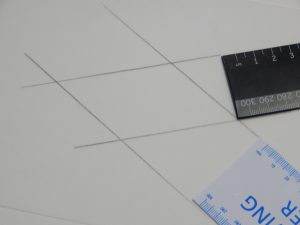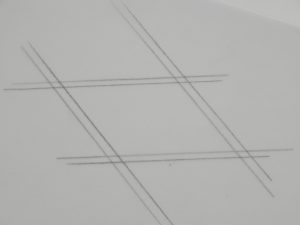We take it that you know that in a parallelogram both pairs of opposite sides are parallel.

In this investigation you will discover some other properties of parallelograms.

Step 1: Use two rulers with different widths to draw a parallelogram on tracing paper or baking paper.

Make sure that it is not a rhombus and that the adjacent sides are not equal in length.Step 2: Place a second piece of tracing paper over the first and copy the parallelogram.

Step 3: Slide the second tracing paper over the first to compare the lengths of the opposite sides of the parallelogram.

How do the lengths of the opposite sides compare?

Now make your own finding or Conjecture 1: Both pairs of opposite sides of a parallelogram are ….

Step 4: Rotate or flip the second paper to compare the sizes of the opposite angles of the parallelogram.

How do the sizes of the opposite angles compare?

Now make your own finding or Conjecture 2: Both pairs of opposite angles of a parallelogram are ….

What can you discover about the point of intersection of the diagonals?

Step 5: Draw or fold the two diagonals of the parallelogram. Place a dot on their intersection.

Step 6: Fold to compare the lengths of the two segments on each diagonal.

Your next finding or Conjecture 3 could be:

The point of intersection of the diagonals of a parallelogram ……

(Idea taken from:  Michael Serra, “Patty Paper Geometry”, Key Curriculum Press, 1994, pp 88 – 89).